/
/
/
11) In the figure, a bar magnet moves away from
Not my Question
Flag Content

# Question : 11) In the figure, a bar magnet moves away from : 2042118

11) In the figure, a bar magnet moves away from the solenoid. The induced current through the resistor R is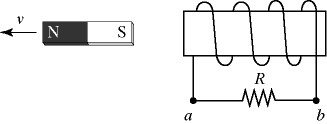A) from a to b.

B) from b to a.

C) There is no induced current through the resistor.

12) In the figure, a copper bar is in contact with a pair of parallel metal rails and is in motion with velocity ν. A uniform magnetic field is present pointing downward, as shown. The bar, the rails, and the resistor R are all in the same plane. The induced current through the resistor R is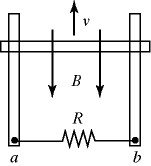A) from a to b.

B) from b to a.

C) There is no induced current through the resistor.

13) In the figure, two parallel wires carry currents of magnitude I in opposite directions. A rectangular loop is midway between the wires. The current I is decreasing with time. The induced current through the resistor R is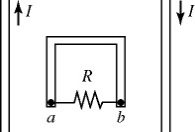A) from a to b.

B) from b to a.

C) There is no induced current through the resistor.

14) A bar magnet is held vertically with its upper end a little bit below the center of a horizontal metal ring. The upper end of the magnet is its north pole, as shown in the figure. The bar magnet is now dropped. An observer views the ring from above its center. To this observer, how will the induced current in the ring behave as the magnet falls?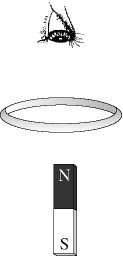A) The current will flow clockwise and be increasing.

B) The current will flow clockwise and be decreasing.

C) The current will flow counter-clockwise and be increasing.

D) The current will flow counter-clockwise and be decreasing.

E) The induced current will be zero.

15) In the figure, two solenoids are side by side. The switch S, initially open, is closed. The induced current through the resistor R is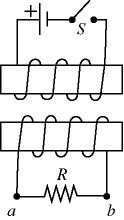A) from a to b.

B) from b to a.

C) There is no induced current through the resistor.

16) In the figure, two solenoids are approaching each other with speed v as shown. The induced current through the resistor R is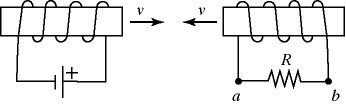A) from a to b.

B) from b to a.

C) There is no induced current through the resistor.

17) In the figure, a straight wire carries a current I. The wire passes through the center of a toroidal coil. If the current in the wire is quickly reduced to zero, the induced current through the resistor R is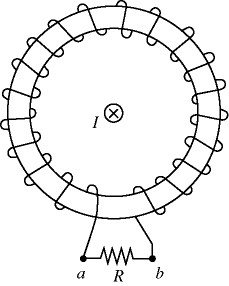A) from a to b.

B) from b to a.

C) There is no induced current through the resistor.

18) In the figure, a C-shaped conductor is in a uniform magnetic field B, which is increasing. The polarity of the induced emf in terminals X and Y is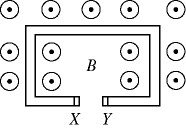A) X and Y are at the same potential.

B) X is positive and Y is negative.

C) X is negative and Y is positive.

19) The figure shows three metal coils labeled A, B, and C heading towards a region where a uniform static magnetic field exists. The coils move with the same constant velocity and all have the same resistance. Their relative sizes are indicated by the background grid. As they enter the magnetic field the coils will have an induced electric current in them. For which coil will the current be the greatest?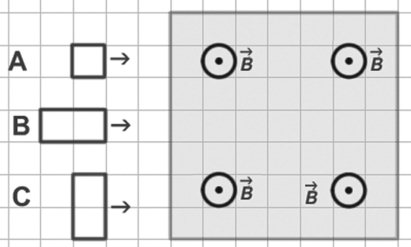A) A

B) B

C) C

D) The current is the same in all three cases since all the coils move with the same velocity.

E) There is no induced current in any of the coils since they move at constant velocity.

20) A capacitor is charging in a simple RC circuit with a dc battery. Which one of the following statements about this capacitor is accurate?

A) There is a magnetic field between the capacitor plates because charge travels between the plates by jumping from one plate to the other.

B) There is no magnetic field between the capacitor plates because no charge travels between the plates.

C) There is a magnetic field between the capacitor plates, even though no charge travels between them, because the magnetic flux between the plates is changing.

D) There is a magnetic field between the capacitor plates, even though no charge travels between them, because the electric flux between the plates is changing.

E) The magnetic field between the capacitor plates is increasing with time because the charge on the plates is increasing.

21) A resistor and an ideal inductor are connected in series to an ideal battery having a constant terminal voltage V0. At the moment contact is made with the battery,

(a) the voltage across the resistor is

A) V0.

B) V0/e.

C) V0/2.

D) zero.

(b) the voltage across the inductor is

A) V0.

B) V0/e.

C) V0/2.

D) zero.

22) Which of the following statements about inductors are correct? There may be more than one correct choice.

A) When it is connected in a circuit, an inductor always resists having current flow through it.

B) Inductors store energy by building up charge.

C) When an inductor and a resistor are connected in series with a DC battery, the current in the circuit is reduced to zero in one time constant.

D) An inductor always resists any change in the current through it.

E) When an inductor and a resistor are connected in series with a DC battery, the current in the circuit is zero after a very long time.

## Solution 5 (1 Ratings )

Solved
Physics 2 Days Ago 79 Views